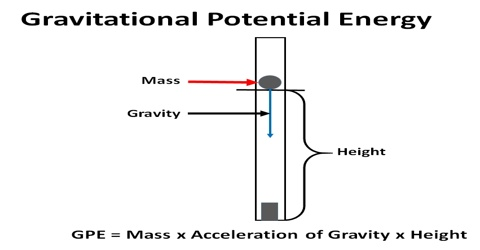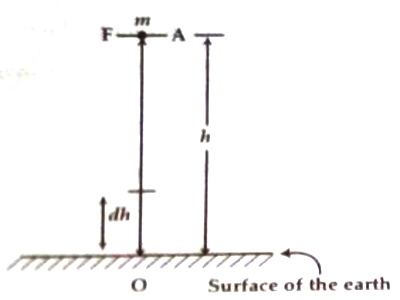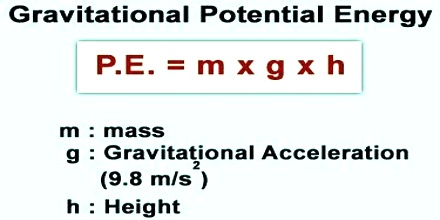Physics

# Gravitational Potential EnergyGravitational Potential energy

To raise a body above the ground against the gravitational force an external source or agent is needed to do work. This work remains stored in the body as potential energy. This energy is called gravitational potential energy. In this case, surface of the earth is considered as the reference level. Let us now measure the potential energy.Calculus method:

Gravitational potential energy is energy an object possesses because of its position in a gravitational field. The most common use of gravitational potential energy is for an object near the surface of the Earth where the gravitational acceleration can be assumed to be constant at about 9.8 m/s2.

Let an object of mass m be raised above the surface of the earth to a height ‘dh’ against the force due to gravity.

Fig: A1

Work done due to this is,

dW = F . dh

or, dW = Fdh … … … (1)

Here, F = force applied by external source, and dh = vertical height. The angle between F and dh is zero.

In order to raise a body above, a vertical force equal to the weight of the body is to be applied.

So, Applied force, F = Weight of the body = mg

So, total work done in raising the body to a height h at position A [Figure] is the summation of the small work done as an equation (1).

Then, Gravitational potential energy = total work done in raising the body to the height h above the ground

so, P. E. = h0 Fdh = h0 mg dh

For small height g is constant (assumed) and we can write,

P.E. = mg h0 dh = mg [h]h0 = mg (h-0) = mgh

So, P.E. = mgh

i.e., Gravitational potential energy = mass x acceleration due to gravity x height above the reference level.As the body descends h becomes less and less, so also the gravitational potential energy. On the surface of the earth, h = 0, the potential energy also becomes zero.

It is to be mentioned that we can never measure the absolute value of potential energy; we can only measure the change in potential energy with respect to a reference or standard level. If the reference level is the ground, then the potential energy at a certain height above it will not be the same if we consider the top of a hill as a reference level.

Potential energy can either be positive or negative. It depends on the reference level. If the reference level is the ground, then at a point above the ground it will be positive. On the other hand inside a mine or inside the earth potential energy is negative.